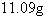Name:    Chapter 5 Post Test

1.

Select the graph of the function.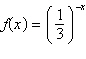a.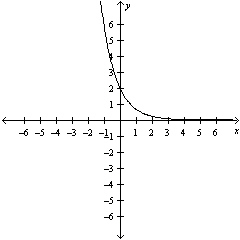d.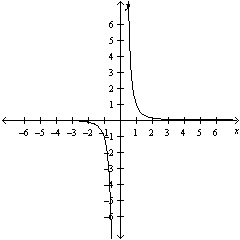b.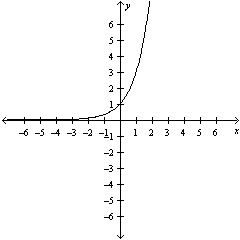e.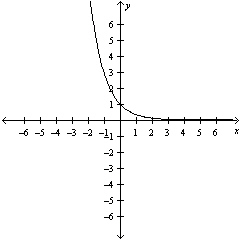c.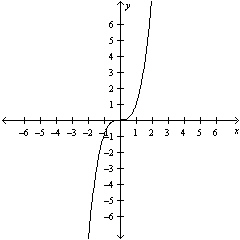2.

Use a graphing utility to construct a table of values for the function. Round your answer to two decimal places.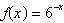a.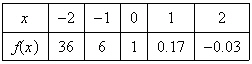b.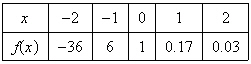c.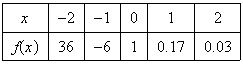d.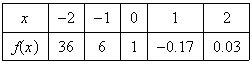e.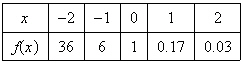3.

Sketch the graph of the function.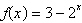a.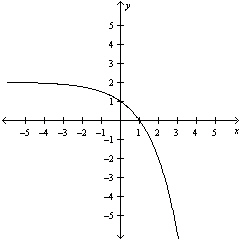d.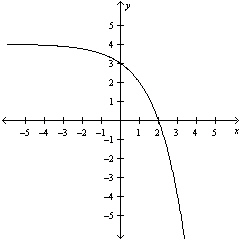b.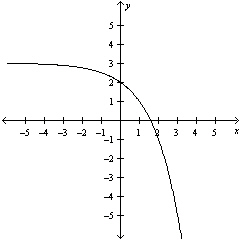e.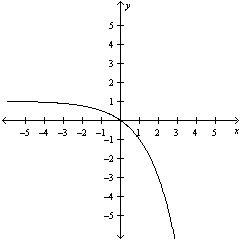c.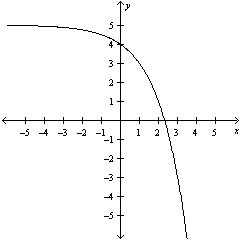4.

Write the exponential equation in logarithmic form.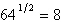a.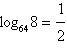b.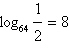c.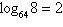d.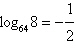e.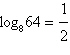5.

Write the exponential equation in logarithmic form.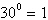a.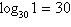b.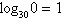c.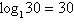d.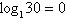e.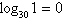6.

Use the One-to-One Property to solve the equation for x.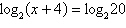a. 19 b. 17 c. 18 d. 16 e. 20

7.

Use the One-to-One Property to solve the equation for x.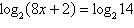a.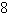b.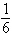c.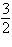d.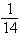e.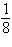8.

Rewrite the logarithm as a ratio of natural logarithms.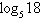a.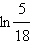b.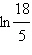c.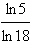d.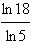e. None of these

9.

Rewrite the logarithm as a ratio of natural logarithms.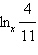a.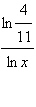b.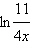c.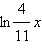d.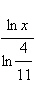e. None of these

10.

Rewrite the logarithm as a ratio of common logarithms.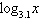a.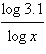b.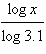c.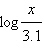d.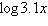e. None of these

11.

Use the change-of-base formula to rewrite the logarithm as a ratio of logarithms. Then use a
graphing utility to graph the ratio.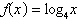a.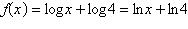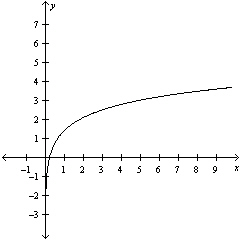d.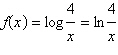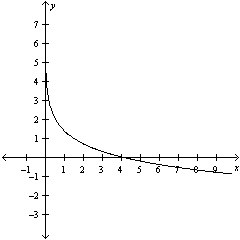b.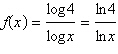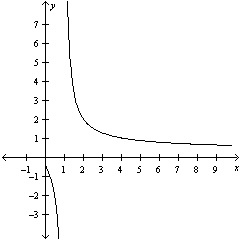e.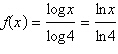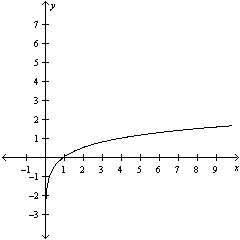c.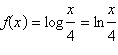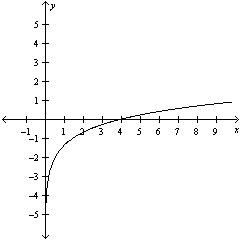12.

Find the exact value of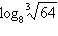without using a calculator.
 a.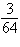b.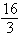c.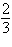d. –1 e.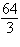13.

Determine whether the given x-value is a solution (or an approximate solution) of the equation.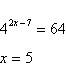a. No b. Yes

14.

Determine whether the given x-value is a solution (or an approximate solution) of the equation.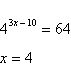a. No b. Yes

15.

Solve for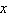.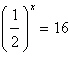a. 2 b.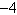c. 6 d. 4 e.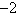16.

Solve for. Approximate the result to three decimal places.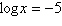a.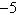b. 0.00001 c.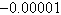d.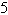e. 1.609

17.

Solve the exponential equation algebraically. Approximate the result to three decimal places.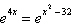a.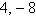b.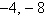c.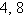d.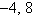e.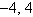18.

\$6500 is invested in an account at interest rate r, compounded continuously. Find the time required for the amount to triple. (Approximate the result to two decimal places.)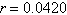a. 15.50 yr b. 14.50 yr c. 26.16 yr d. 18.50 yr e. 17.50 yr

19.

Solve for x: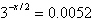. Round to 3 decimal places.
 a. –12.715 b. 9.574 c. 12.715 d. –4.787 e. 10.518

20.

 Isotope Half-life (years) Initial Quantity Amount after 1000 years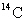5715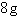----
 a. Amount after 1000 years: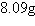b. Amount after 1000 years: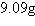c. Amount after 1000 years: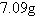d. Amount after 1000 years: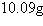e. Amount after 1000 years: